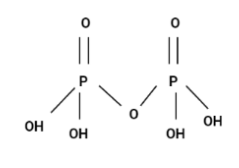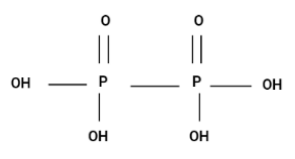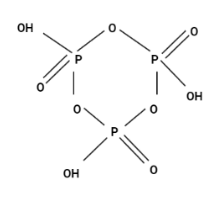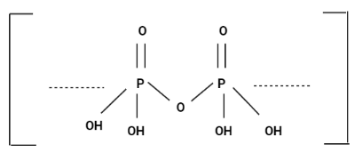Courses
Courses for Kids
Free study material
Offline Centres
MoreLast updated date: 30th Nov 2023
Total views: 279.3k
Views today: 7.79k

# Which of the following is a cyclic oxoacid?(A) ${H_4}{P_2}{O_7}$ (B) ${H_4}{P_2}{O_6}$ (C) ${H_3}{P_3}{O_9}$ (D) ${H_5}{P_5}{O_{15}}$Verified
279.3k+ views
Hint :Oxoacids (also known as oxyacids) are acids that contain oxygen. An oxoacid, to be more specific, is an acid that contains oxygen and contains at least one additional component that has one or more hydrogen atoms bound to oxygen. And cyclic oxoacids is an oxoacid whose chemical structure is cyclic.

Now, to analyse the structure of the compounds first we need to make the chemical structures of all the compounds in the option.
Option (A) ${H_4}{P_2}{O_7}$Since, the structure is a chain and it's not cyclic. Hence, option (A) is incorrect.
Option (B) ${H_4}{P_2}{O_6}$Since, the structure is a chain and it's not cyclic. Hence, option (B) is also incorrect.
Option (C) ${H_3}{P_3}{O_9}$This structure is cyclic oxoacid. Hence, the correct option is (C).
Option (D) ${H_5}{P_5}{O_{15}}$The structure is a chain and it’s not cyclic. This structure is the same as option (C) , the only difference is that this structure is chain instead of cyclic. Hence, option (D) is also incorrect.
Therefore the correct option is (C).

Note :
Oxoacids are acids that contain oxygen as a component. Phosphorus is known to shape a variety of oxoacids, including ${H_3}P{O_4}$ , ${H_3}P{O_3}$ , and others. It is tetrahedrally surrounded by other atoms in phosphorus oxoacids.